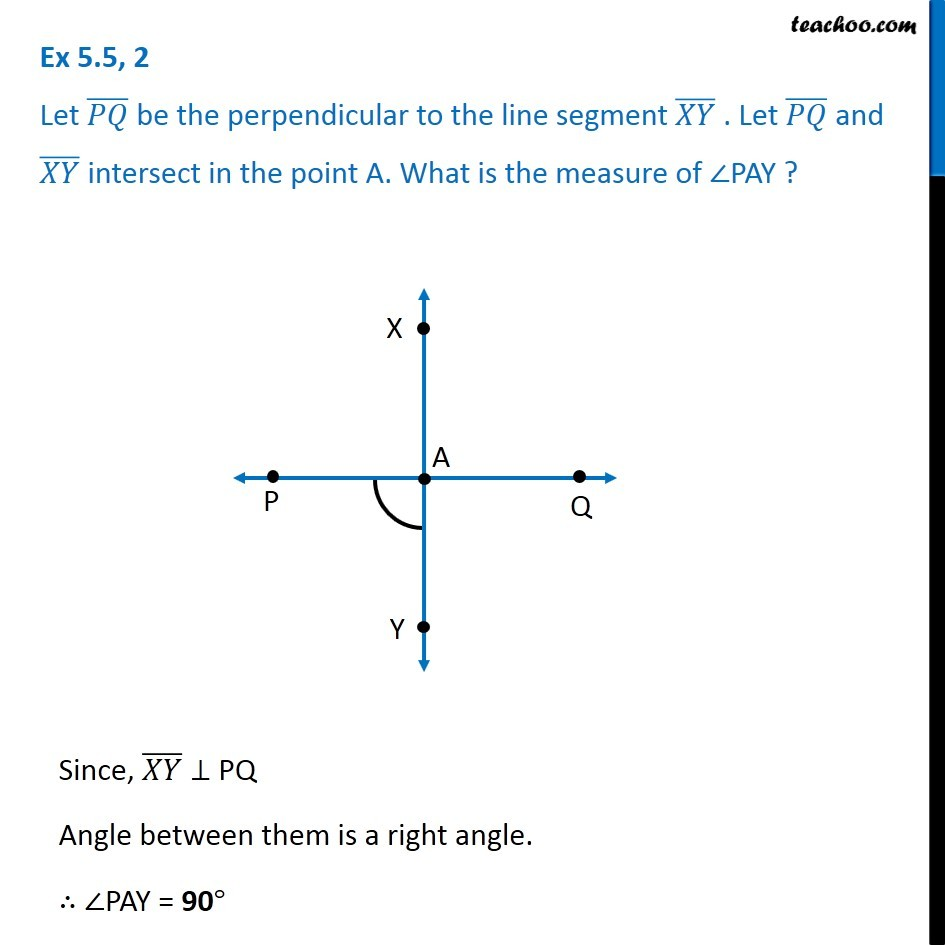1. Chapter 5 Class 6 Understanding Elementary Shapes
2. Concept wise
3. Perpendicular Lines

Transcript

Ex 5.5, 2 Let (𝑃𝑄) ̅ be the perpendicular to the line segment (𝑋𝑌) ̅ . Let (𝑃𝑄) ̅ and (𝑋𝑌) ̅ intersect in the point A. What is the measure of ∠PAY ? Since, (𝑋𝑌) ̅ ⊥ PQ Angle between them is a right angle. ∴ ∠PAY = 90°

Perpendicular Lines